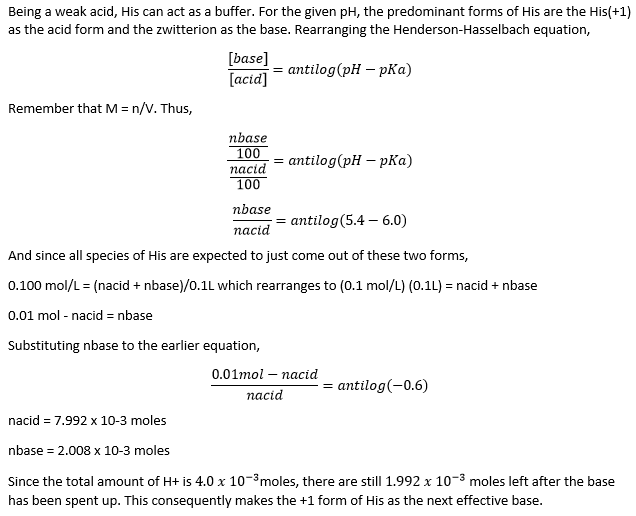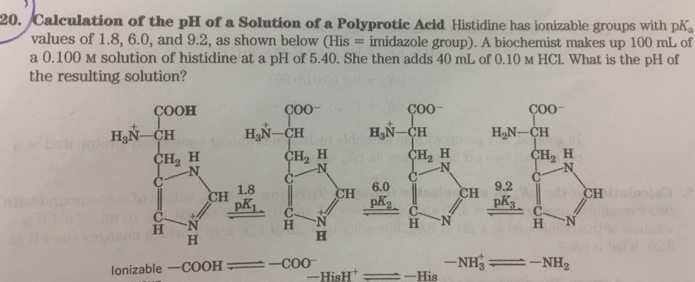# Problem: Calculation of the pH ofaSolution of a Polyprotic AcidHistidine has ionizable groups with pKa values of 1.8, 6.0, and 9.2, as shown below (His = imidazole group). A biochemist makes up 100mL of a 0.100M solution of histidine at a pH of 5.40. She then adds 40mL of 0.10M HCl. What is the pH of the resulting solution?

###### FREE Expert Solution###### Problem Details

Calculation of the pH ofaSolution of a Polyprotic Acid

Histidine has ionizable groups with pKa values of 1.8, 6.0, and 9.2, as shown below (His = imidazole group). A biochemist makes up 100mL of a 0.100M solution of histidine at a pH of 5.40. She then adds 40mL of 0.10M HCl. What is the pH of the resulting solution?